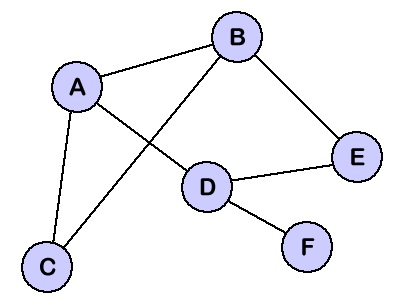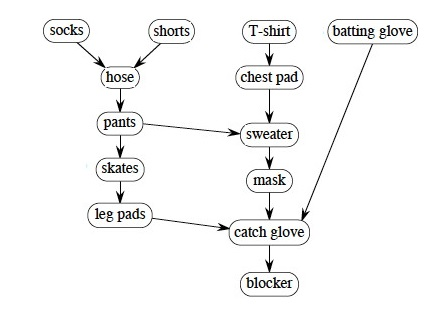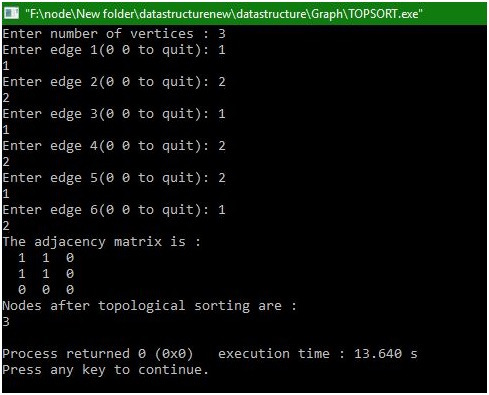# Topological sorting

Submitted by Manu Jemini, on January 12, 2018

A graph is a set of nodes or known number of vertices. When these vertices are paired together, we call it edges. An Edge is a line from one node to other. Every edge can have its cost or weight. Graphs are two types Directed and Undirected. Directed graphs are the graphs in which the vertices are ordered and in undirected graphs the vertices are unordered.Image source: https://courses.cs.vt.edu/csonline/DataStructures/Lessons/Graphs/graph.gif

Node is a vertex in the graph at a position. The Line between two nodes is an edge. The Edge can have weight or cost associated with it. Edge is the line connecting two nodes or a pair of nodes.

A Topological sort with a direct graph of linear ordering with nodes for every direct edge AB from node A to node B, A comes before B when ordering of a directed graph is a linear ordering of its nodes such that for every. A topological order possible only if the graph has no directed cycles, it means, if it is a directed acyclic graph.Image source: http://www2.hawaii.edu/~nodari/teaching/f15/Notes/Topic-14/DAG-topological-sort-example-1.jpg

C program:

```#include<stdio.h>
#define MAX 20

int front=-1,rear=-1,queue[MAX];

void create_graph()
{
int i,max_edges,origin,destin;

printf("Enter number of vertices : ");
scanf("%d",&n);
max_edges=n*(n-1);

for(i=1;i<=max_edges;i++)
{
printf("Enter edge %d(0 0 to quit): ",i);
scanf("%d %d",&origin,&destin);

if((origin==0) && (destin==0))
break;

if( origin > n || destin > n || origin<=0 || destin<=0)
{
printf("Invalid edge!\n");
i--;
}
else
}/*End of for*/
}/*End of create_graph()*/

void display()
{
int i,j;
for(i=1;i<=n;i++)
{
for(j=1;j<=n;j++)
printf("\n");
}
}/*End of display()*/

void insert_queue(int node)
{
if (rear==MAX-1)
printf("Queue Overflow\n");
else
{
if (front==-1)  /*If queue is initially empty */
front=0;
rear=rear+1;
queue[rear] = node ;
}
}/*End of insert_queue()*/

int delete_queue()
{
int del_item;
if (front == -1 || front > rear)
{
printf("Queue Underflow\n");
return 0;
}
else
{
del_item=queue[front];
front=front+1;
return del_item;
}
}/*End of delete_queue() */

int indegree(int node)
{
int i,in_deg=0;
for(i=1;i<=n;i++)
in_deg++;
return in_deg;
}/*End of indegree() */

main()
{
int i,j=0,k;
int topsort[MAX],indeg[MAX];

create_graph();
display();
/*Find the indegree of each node*/
for(i=1;i<=n;i++)
{
indeg[i]=indegree(i);
if( indeg[i]==0 )
insert_queue(i);
}

while(front<=rear) /*Loop till queue is not empty */
{
k=delete_queue();
topsort[j++]=k; /*Add node k to topsort array*/
/*Delete all edges going fron node k */
for(i=1;i<=n;i++)
{
{
indeg[i]=indeg[i]-1;
if(indeg[i]==0)
insert_queue(i);
}
}/*End of for*/
}/*End of while*/

printf("Nodes after topological sorting are :\n");
for(i=0;i<j;i++)
printf( "%d ",topsort[i] );
printf("\n");
}/*End of main()*/
```

OutputPreparation

What's New

Top Interview Coding Problems/Challenges!